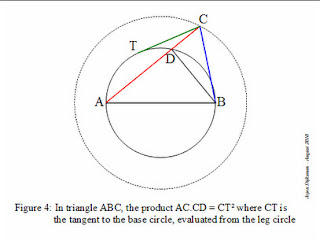## Monday, 16 August 2010

### Another "Almost Pythagorean" RelationshipA while back Arjen Dijksman at "Physics Intuitions" posted a blog reflecting on my post about Almost Pythagorean Triangles . Then he went me one better with a new relation that is pretty incredible. In essence, he shows that for ANY triangle, ABC with opposite sides a, b, and c, there is a relation a2+b2=c2+2 t2, where t length of the tangent from point C to the circle with diameter AB.

He has some nice clear graphics to show the development.. check it out..and with a change of sign the equation generalizes to triangles where c lies inside the circle with diameter AB.

This is, of course, too close to the law of cosines to be ignored.. and it is easy to see that t2 = a*b*cos(C)... in fact as soon as we set t2=AC*DC, and recognize that angle BDC is a right angle, we have Cos(C)= DC/BC... thus AC*DC = AC*BC*(DC/BC)= ab Cos(C).

#### 1 comment:

jason.t.stein said...

I always teach the law of cosines as "Every triangle is almost a right triangle." Students remember the formula better.